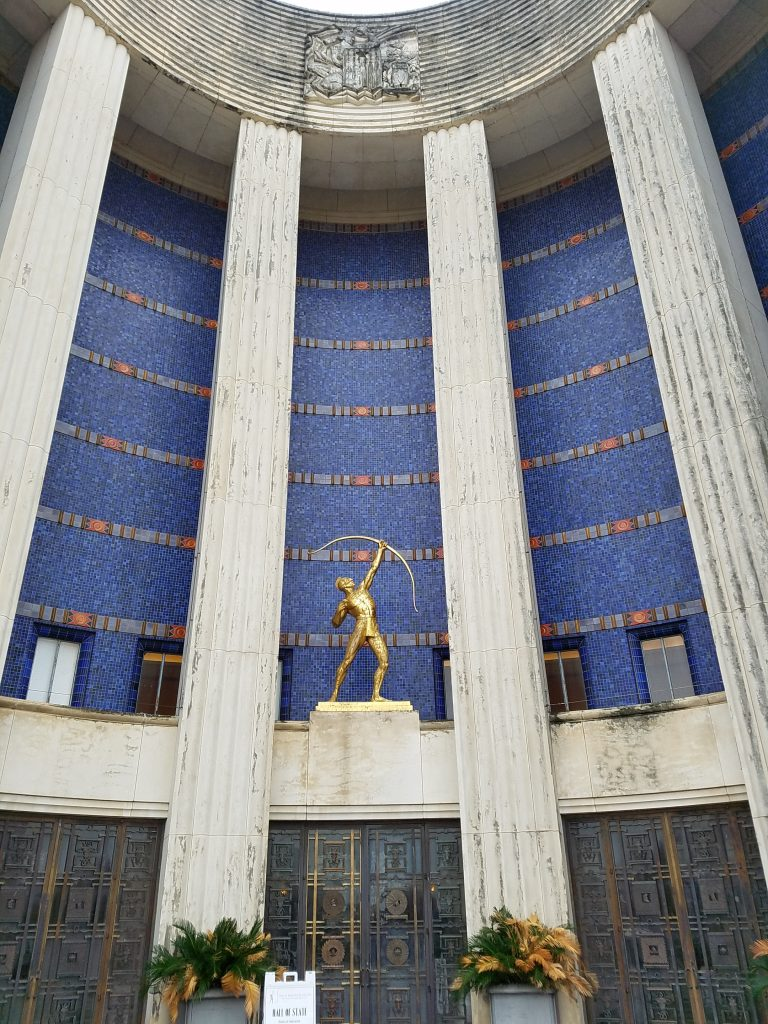08 Mar

The facade of the Hall of State at Dallas Fair Park is awash in small blue tiles.As such, it provides an excellent opportunity for a fun element of almost any math walk: A Really Big Number. In this case, how many tiles are there on the facade?

To get a handle on this, we will take advantage of the fact that the tiles are in uniform horizontal rows. So we just need to estimate how many tiles in each row and how many rows there are in all, and then multiply.

For the first estimate, notice that the facade is shaped like a giant cylinder standing on its circular base; or rather, it’s a semi-cylinder, with horizontal rows of tiles along the semicircular arc of its perimeter. Go and stand where you think the center of that semicircular arc is.

The center of a semicircle is on its diameter, halfway between its endpoints where it meets the arc. So to find that position, you can count the flagstones horizontally between the two ends of the semicircular arc of the facade. There are 13 flagstones across, so the center is at the midpoint of the center (7th) flagstone.

But what we really want is the perimeter of that semicircle. Dividing the usual formula defining pi by two, we get that the length of a semicircle is pi times the radius of the semicircle (since the radius is half of the diameter). Measuring the flagstones, you will find that they are 40 inches across by 43 inches deep (toward the building). But now don’t be fooled! Even though there are six and a half flagstones from the center to the wall of the building on the left and right, that’s not the radius of the semicircle formed by a row of blue tiles — there is a balcony above the doors to the building, and the blue wall of tiles is behind that balcony. So don’t just multiply 40 inches by 6.5 to get the radius.

Instead, we will take the measurement from the center straight ahead to the inside doors of the building, which we can guess are directly below the blue wall of tiles (guessing that the compartment between the inner and outer doors on the ground level is the same depth as the balcony above, because architects like to line things up neatly). And that turns out to be six of the long dimensions of the flagstones, plus 38 inches for the last partial flagstone that lines things up with the building, plus eight feet to the inner doors, for a total of 6×43 + 38 + 96 = 392 inches.

Multiplying by pi, each row of tiles is approximately 1,232 inches long. Next, we need to know how big each tile is. Most likely, they are squares a whole number of inches on a side. So to get an idea of how many inches, take a look at how many tiles tall those windows on the second level are: about 24 tiles tall. So are the windows most likely to be two feet, four feet, or six feet tall? Our experience tells us that a two-foot window would be quite short and a six-foot window would be unusually tall (especially for a building built in 1936), so we conclude the tiles are two inches by two inches.

Therefore, dividing the perimeter of the semicircle by 2, there are approximately 616 tiles in one horizontal row. To count how many rows, note that the tiles are in seven groups vertically, and we can simply count that there are 30 rows of tiles in each group, for 210 rows in all. So, ignoring the tiles missing because of the windows, there are roughly 616×210 = 129,360 blue tiles on the front of the Hall of State!